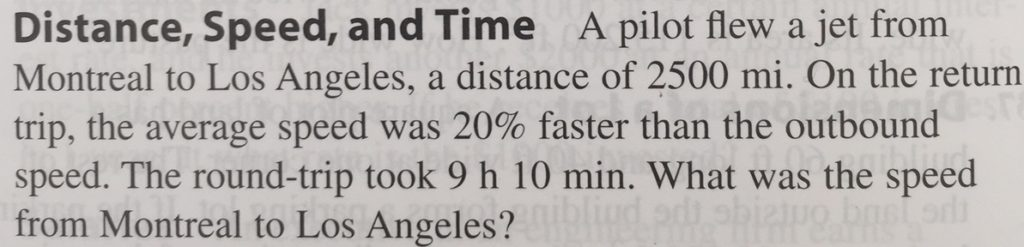## Distance and Speed Math Word Problems

Word ProblemsIf it is confusing at first, then try to make a similar problem that is simpler.

For this one, let us start with a plane traveling a distance of 1000 miles and it takes 5 hours, and we want to find the speed.

First, draw a diagram.

Plane ->

A———————————————B

1000 Miles

For this type of problems you usually deal with rates.

Think of a car. You usually say something like 20 miles per hour, which is

${20}\frac{miles\left(distance\right)}{hour\left(time\right)}$

We have three items we can work with here: 1000 miles, 5

hours, and

${x}\frac{miles}{hour}$

We can begin by experimenting.

We get something weird here, miles squared. We want to try to cancel out the unit measures (miles and hours here) so that they are equal on both sides.

We made use of this property:

The rate is 200 miles per hour.

We could have made the initial problem even simpler. Make the distance 1000 miles, the time 1 hour, and the rate would just be 1000 miles per hour.

We can now make a slightly more complex problem that is closer to the original.

Let us say the plane now returns from it trip and it travels for a total of 2000 miles in 10 hours.

First, draw a diagram.

First Trip -> 1000 Miles

A————————————————————B

1000 Miles <- Return Trip

1000 Miles

I will not use the units in the equation for now, since we checked that it works out before.

$\frac{1000}{\mathrm{x}}{+}\frac{1000}{\mathrm{x}}{=}{10}$

$\frac{2000}{x}{=}{10}$

${x}{=}{200}$

The rate is still 200 miles per hour, which makes sense since we made a return trip in the same amount of time.

Now, we know how things work, and can solve the original problem.

First, draw a diagram.

First Trip -> Speed of X

A————————————————————B

Speed of 1.2X <- Return Trip

2500 Miles Each way

Convert the 10 minutes to hours: 10/60 = 1/6

$\frac{2500}{x}{+}\frac{2500}{1.2x}{=}{9}{+}\frac{1}{6}$

LCD = 1.2x

${1}{.}{2}{x}{\left(}\frac{2500}{x}{\right)}{+}{1}{.}{2}{x}{\left(}\frac{2500}{1.2x}{\right)}{=}{1}{.}{2}{\left(}{9}{+}\frac{1}{6}{\right)}$

${3000}{+}{2500}{=}{1}{.}{2}{x}{\left(}{9}{+}\frac{1}{6}{\right)}$

${5500}{=}{1}{.}{2}{x}{\left(}{9}{+}\frac{1}{6}{\right)}$

${5500}{\left(}\frac{1}{\left(1.2\right)\left(9+\frac{1}{6}\right)}{\right)}{=}{x}{\left(}{1}{.}{2}{\right)}{\left(}{9}{+}\frac{1}{6}{\right)}{\left(}\frac{1}{\left(1.2\right)\left(9+\frac{1}{6}\right)}{\right)}$

${\left(}\frac{5500}{\left(1.2\right)\left(9+\frac{1}{6}\right)}{\right)}{=}{x}$

Use a calculator.

x = 500 miles per hour

If you ever encounter a difficult problem, then try to make a simpler problem that mimics the style of the original question. Remember, you can make the numbers as easy as you want. Play around with it, and slowly build up from there until you understand the underlying concepts. Lastly and hopefully, you should be able to tackle the more difficult problem.